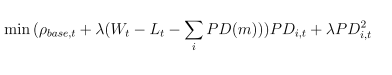# I have an error on my code " Invalid quadratic form(s): not a square."

I’m working to minimize a cost function , I want to exclude one element from a summation " it’s sounds like my Dvar will affect them and vice versa

this the problem that I’m working onthis my code so far ,

`````` for i = 1:I
for t = 1:T
cvx_begin
variable  pd(I,T) ;
f1 = lamda_base .*pd(i,t) + beta .* pd(i,t).^2;
f2 = beta *(L(t)-w(t)+ sum(pd(1:i-1,t))- sum(pd(i+1:I,t))).*pd(i,t);
minimize (f1+f2)
``````

I really appreciate your help , I believe that the problem in the part which relate to excluding one element from the summation , but I can’t figure it out

You haven’t followed CVX’s rules, and therefore have not convinced CVX that your objective function is convex. Can you formulate all quadratic and cross-terms in pd (i.e., everything other than linear terms) in the form
`pd' * A * pd`
where A is positive semi-definite? If so, you should be able to enter the model such that CVX wil accept it. Then you need to make sure it matches what you really want to do, which is far from obvious to me. Among other things, do you really mean to be solving T * I separate optimization (CVX) problems?